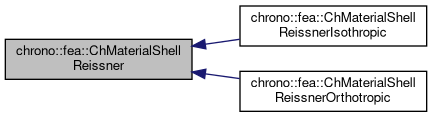chrono::fea::ChMaterialShellReissner Class Reference

## Description

Material for a single layer of a 6-field Reissner-Mindlin shells (kinematically-exact shell theory as in Witkowski et al).

This base implementation assumes that one creates a ChMaterialShellReissner by providing three components:

Thickness is defined when adding a ChMaterialShellReissner material as a layer in a shell finite element (ex. ChElementShellReissner4). A material can be shared between multiple layers.

#include <ChMaterialShellReissner.h>

Inheritance diagram for chrono::fea::ChMaterialShellReissner:[legend]

## Public Member Functions

ChMaterialShellReissner (std::shared_ptr< ChElasticityReissner > melasticity)

ChMaterialShellReissner (std::shared_ptr< ChElasticityReissner > melasticity, std::shared_ptr< ChPlasticityReissner > mplasticity)

ChMaterialShellReissner (std::shared_ptr< ChElasticityReissner > melasticity, std::shared_ptr< ChPlasticityReissner > mplasticity, std::shared_ptr< ChDampingReissner > mdamping)

virtual void ComputeStress (ChVector<> &n_u, ChVector<> &n_v, ChVector<> &m_u, ChVector<> &m_v, const ChVector<> &eps_u, const ChVector<> &eps_v, const ChVector<> &kur_u, const ChVector<> &kur_v, const double z_inf, const double z_sup, const double angle, ChShellReissnerInternalData *mdata_new=nullptr, const ChShellReissnerInternalData *mdata=nullptr)
Compute the generalized cut force and cut torque, given the actual generalized section strain expressed as deformation vector e and curvature k, that is: {n_u,n_v,m_u,m_v}=f({e_u,e_v,k_u,k_v}), and given the actual material state required for plasticity if any (but if mdata=nullptr, computes only the elastic force). More...

virtual void ComputeStiffnessMatrix (ChMatrixRef K, const ChVector<> &eps_u, const ChVector<> &eps_v, const ChVector<> &kur_u, const ChVector<> &kur_v, const double z_inf, const double z_sup, const double angle, const ChShellReissnerInternalData *mdata=nullptr)
Compute the 6x6 tangent material stiffness matrix [Km] = dσ/dε at a given strain state, and at given internal data state (if mdata=nullptr, computes only the elastic tangent stiffenss, regardless of plasticity). More...

void SetElasticity (std::shared_ptr< ChElasticityReissner > melasticity)
Set the elasticity model for this section. More...

std::shared_ptr< ChElasticityReissnerGetElasticity ()
Get the elasticity model for this section. More...

void SetPlasticity (std::shared_ptr< ChPlasticityReissner > mplasticity)
Set the plasticity model for this section. More...

std::shared_ptr< ChPlasticityReissnerGetPlasticity ()
Get the elasticity model for this section, if any. More...

void SetDamping (std::shared_ptr< ChDampingReissner > mdamping)
Set the damping model for this section. More...

std::shared_ptr< ChDampingReissnerGetDamping ()
Get the damping model for this section. More...

void SetDensity (double md)
Set the density of the shell (kg/m^3)

double GetDensity () const

double Get_rho () const
for backward compatibility - use GetDensity instead

## ◆ ChMaterialShellReissner() [1/3]

 chrono::fea::ChMaterialShellReissner::ChMaterialShellReissner ( std::shared_ptr< ChElasticityReissner > melasticity )
Parameters
 melasticity elasticity model

## ◆ ChMaterialShellReissner() [2/3]

 chrono::fea::ChMaterialShellReissner::ChMaterialShellReissner ( std::shared_ptr< ChElasticityReissner > melasticity, std::shared_ptr< ChPlasticityReissner > mplasticity )
Parameters
 melasticity elasticity model mplasticity plasticity model, if any

## ◆ ChMaterialShellReissner() [3/3]

 chrono::fea::ChMaterialShellReissner::ChMaterialShellReissner ( std::shared_ptr< ChElasticityReissner > melasticity, std::shared_ptr< ChPlasticityReissner > mplasticity, std::shared_ptr< ChDampingReissner > mdamping )
Parameters
 melasticity elasticity model mplasticity plasticity model, if any mdamping damping model, if any

## ◆ ComputeStiffnessMatrix()

 void chrono::fea::ChMaterialShellReissner::ComputeStiffnessMatrix ( ChMatrixRef K, const ChVector<> & eps_u, const ChVector<> & eps_v, const ChVector<> & kur_u, const ChVector<> & kur_v, const double z_inf, const double z_sup, const double angle, const ChShellReissnerInternalData * mdata = nullptr )
virtual

Compute the 6x6 tangent material stiffness matrix [Km] = dσ/dε at a given strain state, and at given internal data state (if mdata=nullptr, computes only the elastic tangent stiffenss, regardless of plasticity).

Parameters
 K 12x12 stiffness matrix eps_u strains along u direction eps_v strains along v direction kur_u curvature along u direction kur_v curvature along v direction z_inf layer lower z value (along thickness coord) z_sup layer upper z value (along thickness coord) angle layer angle respect to x (if needed) mdata material internal variables, at this point, if any, including {p_strain_e, p_strain_k, p_strain_acc}

## ◆ ComputeStress()

 void chrono::fea::ChMaterialShellReissner::ComputeStress ( ChVector<> & n_u, ChVector<> & n_v, ChVector<> & m_u, ChVector<> & m_v, const ChVector<> & eps_u, const ChVector<> & eps_v, const ChVector<> & kur_u, const ChVector<> & kur_v, const double z_inf, const double z_sup, const double angle, ChShellReissnerInternalData * mdata_new = nullptr, const ChShellReissnerInternalData * mdata = nullptr )
virtual

Compute the generalized cut force and cut torque, given the actual generalized section strain expressed as deformation vector e and curvature k, that is: {n_u,n_v,m_u,m_v}=f({e_u,e_v,k_u,k_v}), and given the actual material state required for plasticity if any (but if mdata=nullptr, computes only the elastic force).

If there is plasticity, the stress is clamped by automatically performing an implicit return mapping. In sake of generality, if possible this is the function that should be used by beam finite elements to compute internal forces, ex.by some Gauss quadrature.

Parameters
 n_u forces along u direction (per unit length) n_v forces along v direction (per unit length) m_u torques along u direction (per unit length) m_v torques along v direction (per unit length) eps_u strains along u direction eps_v strains along v direction kur_u curvature along u direction kur_v curvature along v direction z_inf layer lower z value (along thickness coord) z_sup layer upper z value (along thickness coord) angle layer angle respect to x (if needed) mdata_new updated material internal variables, at this point, including {p_strain_e, p_strain_k, p_strain_acc} mdata current material internal variables, at this point, including {p_strain_e, p_strain_k, p_strain_acc}

## ◆ GetDamping()

 std::shared_ptr chrono::fea::ChMaterialShellReissner::GetDamping ( )
inline

Get the damping model for this section.

By default no damping.

## ◆ GetElasticity()

 std::shared_ptr chrono::fea::ChMaterialShellReissner::GetElasticity ( )
inline

Get the elasticity model for this section.

Use this function to access parameters such as stiffness, Young modulus, etc. By default it uses a simple centered linear elastic model.

## ◆ GetPlasticity()

 std::shared_ptr chrono::fea::ChMaterialShellReissner::GetPlasticity ( )
inline

Get the elasticity model for this section, if any.

Use this function to access parameters such as yeld limit, etc.

## ◆ SetDamping()

 void chrono::fea::ChMaterialShellReissner::SetDamping ( std::shared_ptr< ChDampingReissner > mdamping )

Set the damping model for this section.

By default no damping.

## ◆ SetElasticity()

 void chrono::fea::ChMaterialShellReissner::SetElasticity ( std::shared_ptr< ChElasticityReissner > melasticity )

Set the elasticity model for this section.

By default it uses a simple centered linear elastic model, but you can set more complex models.

## ◆ SetPlasticity()

 void chrono::fea::ChMaterialShellReissner::SetPlasticity ( std::shared_ptr< ChPlasticityReissner > mplasticity )

Set the plasticity model for this section.

This is independent from the elasticity model. Note that by default there is no plasticity model, so by default plasticity never happens.

The documentation for this class was generated from the following files:
• /builds/uwsbel/chrono/src/chrono/fea/ChMaterialShellReissner.h
• /builds/uwsbel/chrono/src/chrono/fea/ChMaterialShellReissner.cpp